# The NLMIXED Procedure

### Line-Search Methods

In each iteration k, the (dual) quasi-Newton, conjugate gradient, and Newton-Raphson minimization techniques use iterative line-search algorithms that try to optimize a linear, quadratic, or cubic approximation of f along a feasible descent search direction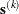,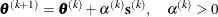by computing an approximately optimal scalar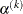.

Therefore, a line-search algorithm is an iterative process that optimizes a nonlinear function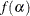of one parameter () within each iteration k of the optimization technique. Since the outside iteration process is based only on the approximation of the objective function, the inside iteration of the line-search algorithm does not have to be perfect. Usually, it is satisfactory that the choice ofsignificantly reduces (in a minimization) the objective function. Criteria often used for termination of line-search algorithms are the Goldstein conditions (see Fletcher 1987).

You can select various line-search algorithms by specifying the LINESEARCH= option. The line-search method LINESEARCH= 2 seems to be superior when function evaluation consumes significantly less computation time than gradient evaluation. Therefore, LINESEARCH= 2 is the default method for Newton-Raphson, (dual) quasi-Newton, and conjugate gradient optimizations.

You can modify the line-search methods LINESEARCH= 2 and LINESEARCH= 3 to be exact line searches by using the LSPRECISION= option and specifying the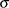parameter described in Fletcher (1987). The line-search methods LINESEARCH= 1, LINESEARCH= 2, and LINESEARCH= 3 satisfy the left-side and right-side Goldstein conditions (see Fletcher 1987). When derivatives are available, the line-search methods LINESEARCH= 6, LINESEARCH= 7, and LINESEARCH= 8 try to satisfy the right-side Goldstein condition; if derivatives are not available, these line-search algorithms use only function calls.